# Varactor Phase Shifters

The varactor phase shifter is another type of quadrature phase shifter, but in this case you are varying the imaginary impedance of the loads (as opposed to the real impedance presented by the PIN diode).

A varactor is a voltage-variable capacitor. Typically you can achieve a range where the capacitance doubles from one voltage extreme to the other, e.g. a varactor could supply between 1 pF and 2 pF. As you probably know, capacitive reactance is calculated by the formula: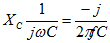The reflection coefficient from a shunt capacitor is simple to calculate: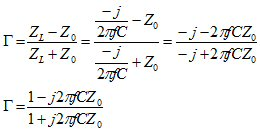Later we'll put this all into as spreadsheet for you. If we were more inclined to work the math, we could probably show how this complex number will always have a value of unity for any value of C or f, and an angle between 0 and -180 degrees.

Now we'll use ADS to create a reflection phase shifter with an ideal coupled-line coupler and a matched pair of capacitors. We added a transmission line with length -90 degrees to "de-embed" the data, which in this case was merely to move the transmission angle away from the 180 degree point to avoid the annoying -180/+180 flip. We used a "parameter sweep" token to drive the capacitors' values from 1 to 100 pF.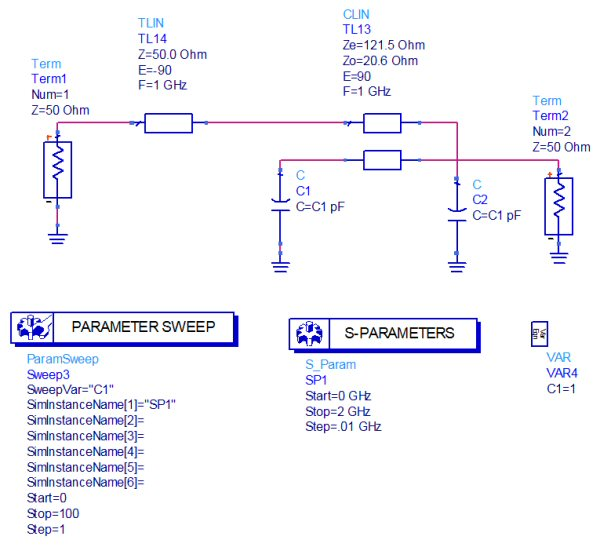Here's the impedance match at all of the capacitor values. If we had solved Zo and Ze for an exact -3.01 dB splitter there would be no variation with capacitor value. The impedance match of -20 dB is provided over almost 60% bandwidth.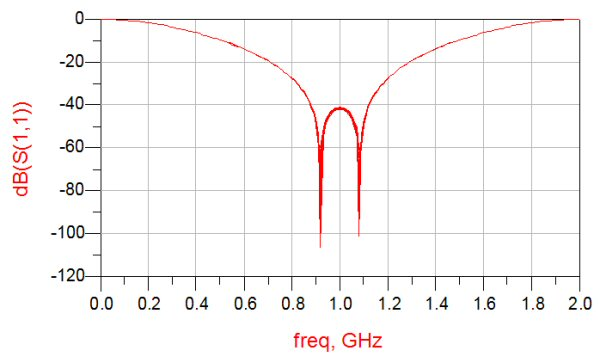Below is the insertion loss of the circuit. It behaves well over a wide band, mostly because a coupled-line coupler provides a perfect quadrature split regardless of frequency. Don't expect the same bandwidth from a branchline coupler!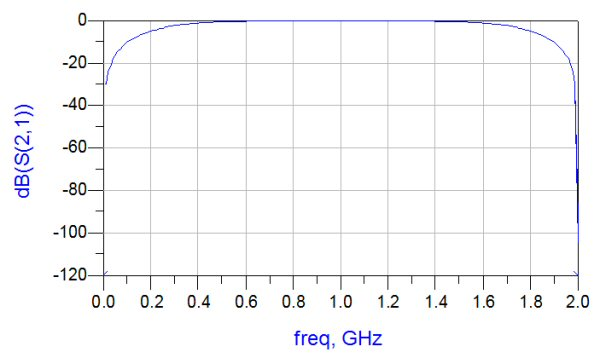Now we'll look at the phase states versus capacitor value. When the capacitors are swept from 0 pF to 100 pF, nearly 180 degrees of phase shift is provided. Indeed, in this type of coupler to get the full 180 degrees you'd need to vary the capacitor from zero to infinity!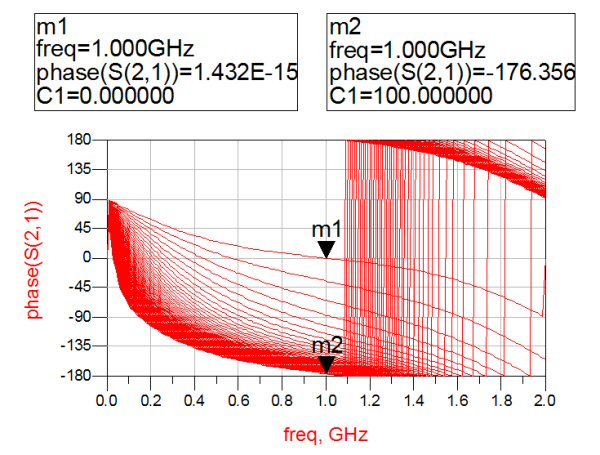Now then, what happens when you only have a 2:1 variation in capacitance? Below we have varied the caps from 2 to 4 pF in steps of 0.2 pF, resulting in less than 40 degrees of phase shift. This is about what you can expect when you design a varactor phase shifter.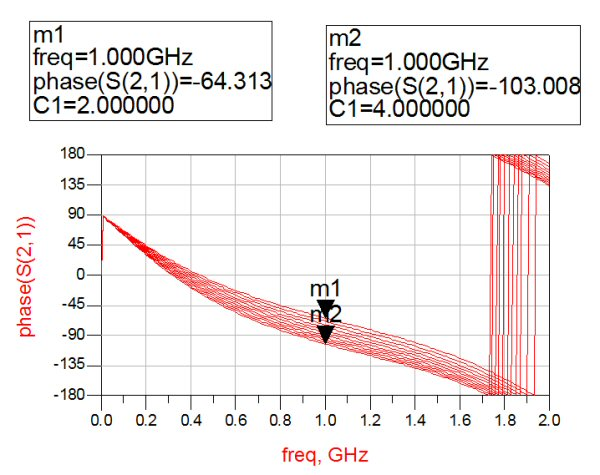Thus varactor phase shifters of this type are used to trim phases that are not very far apart.

What happens when the capacitors inevitably become slightly mismatched? The reflection coefficients at input and output suffer.

Author : Unknown Editor